# RS Aggarwal Solutions for Class 12 Maths Chapter 13: Methods of Integration Exercise 13C

The problems of exercise 13C are based on the concept of integration by parts. The theorem which is explained under this exercise mainly provides an exact idea about the steps to be followed in solving problems. Students can clear their doubts instantly by solving problems using solutions prepared by our experts. It improves the time management skills among students, which are important from the exam point of view. RS Aggarwal Solutions Class 12 Maths Chapter 13 Methods of Integration Exercise 13C PDF links are available below.

## RS Aggarwal Solutions for Class 12 Maths Chapter 13: Methods of Integration Exercise 13C Download PDF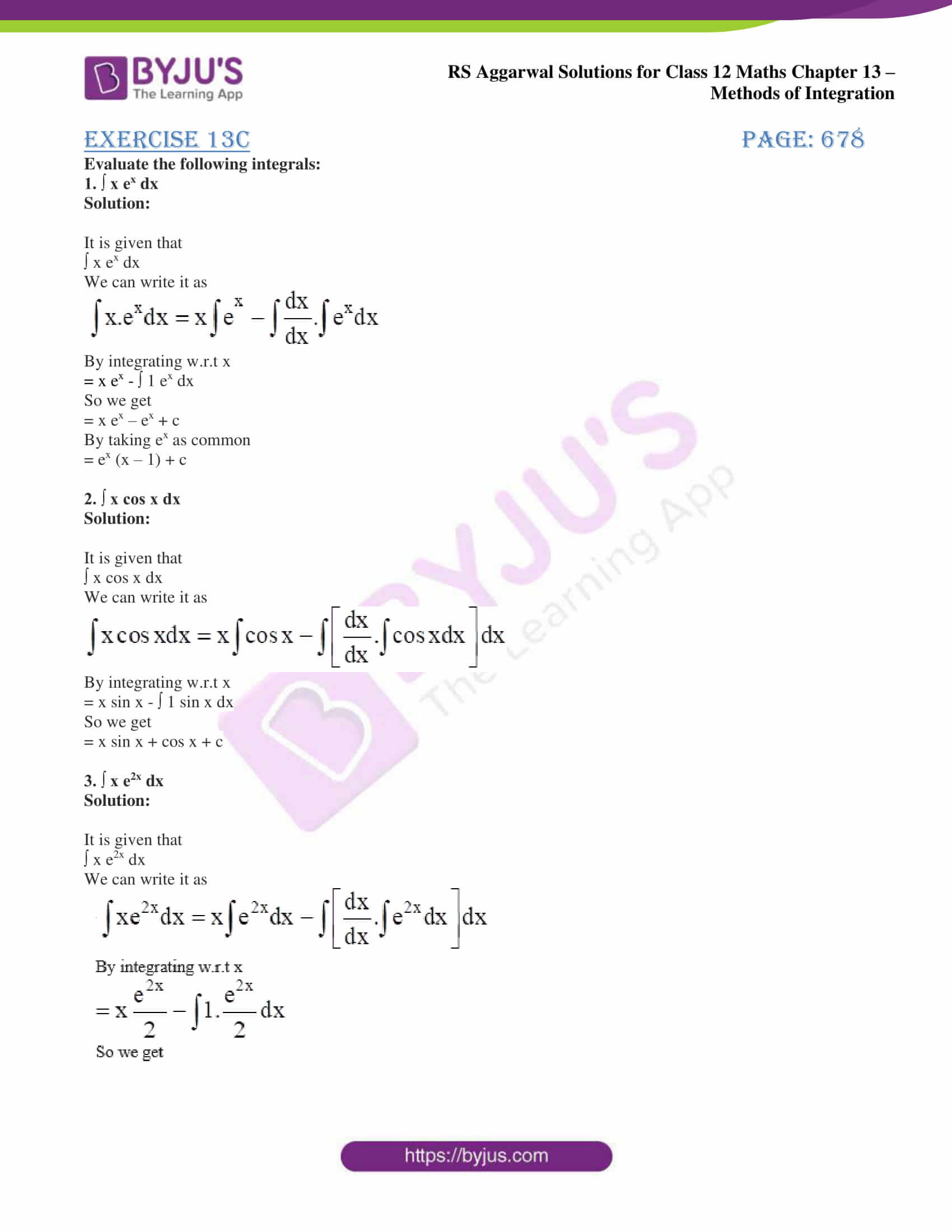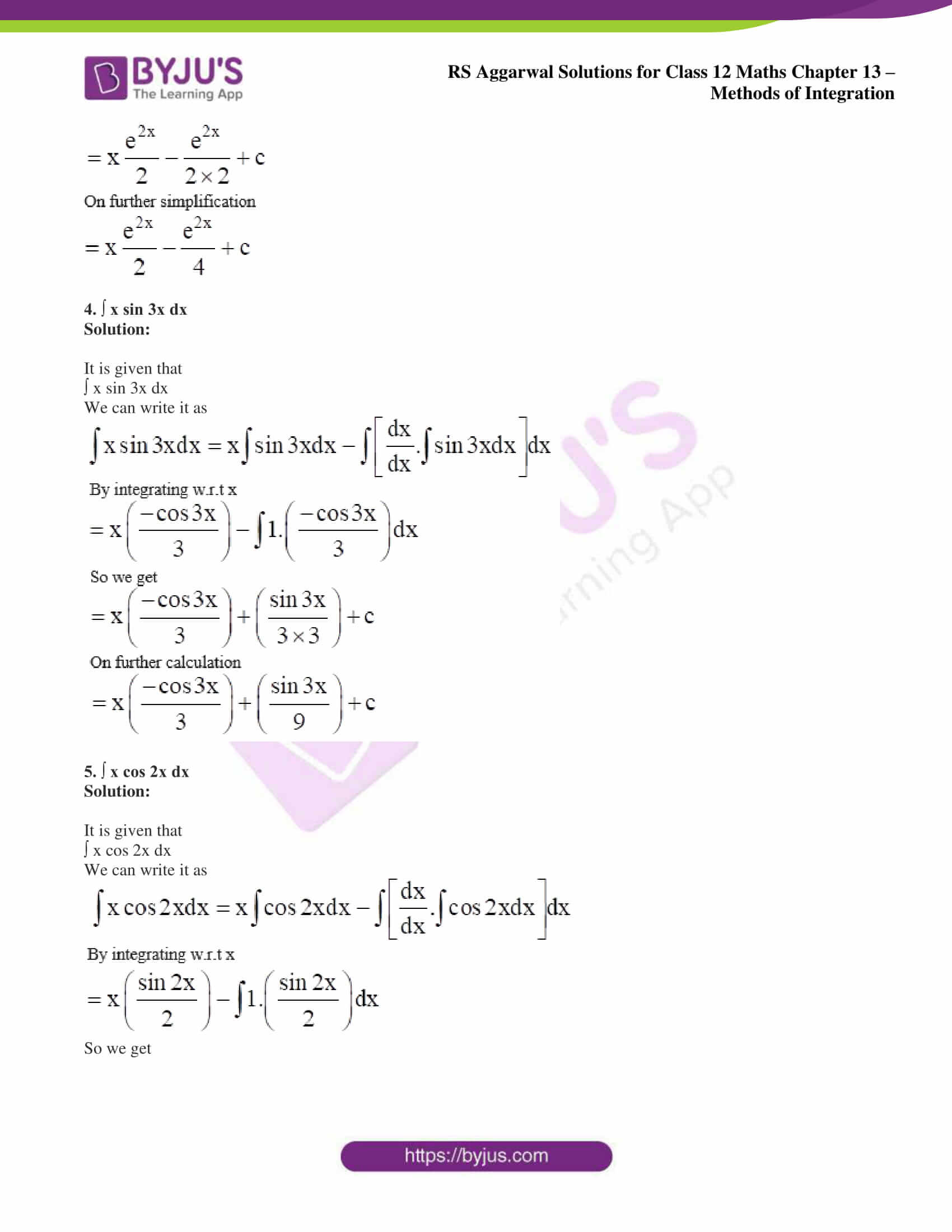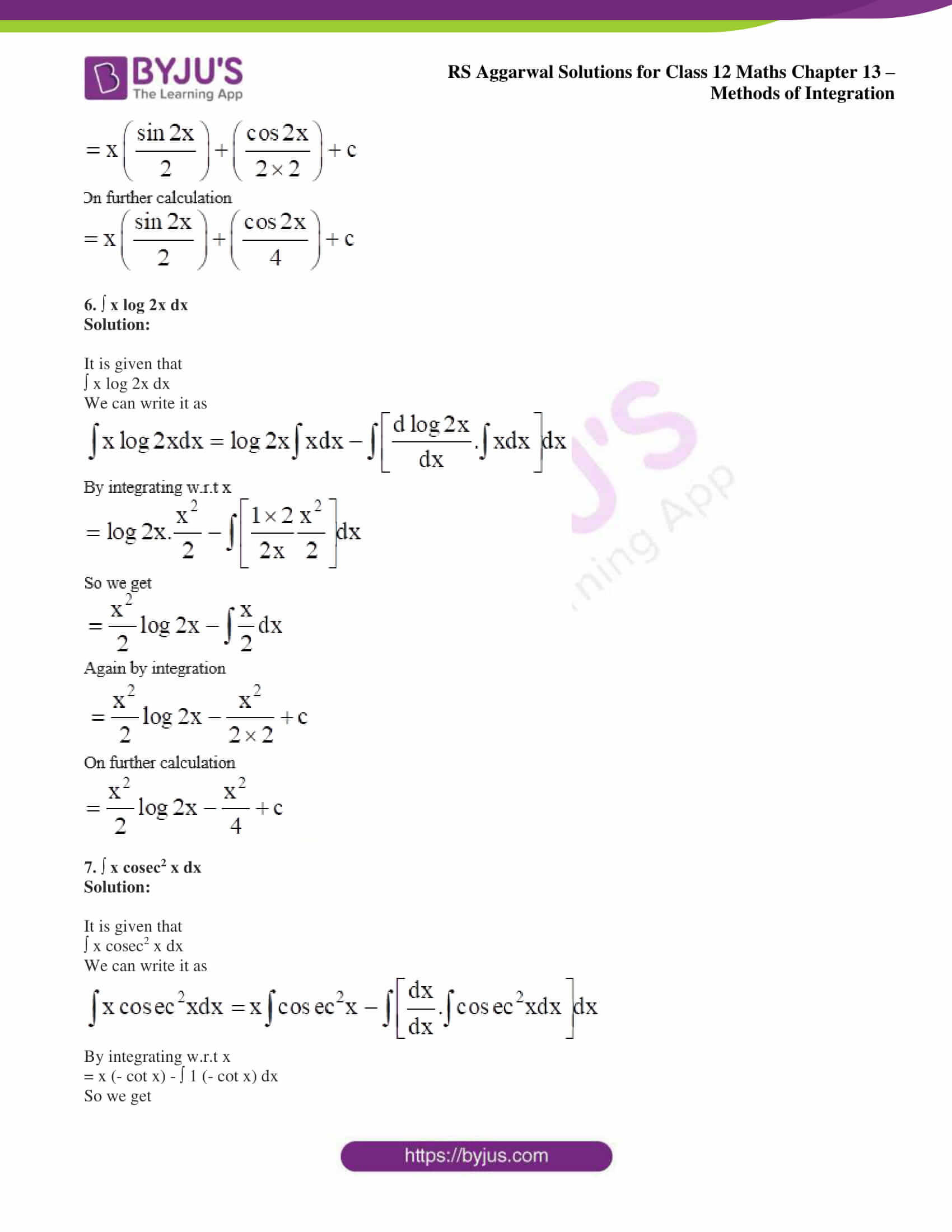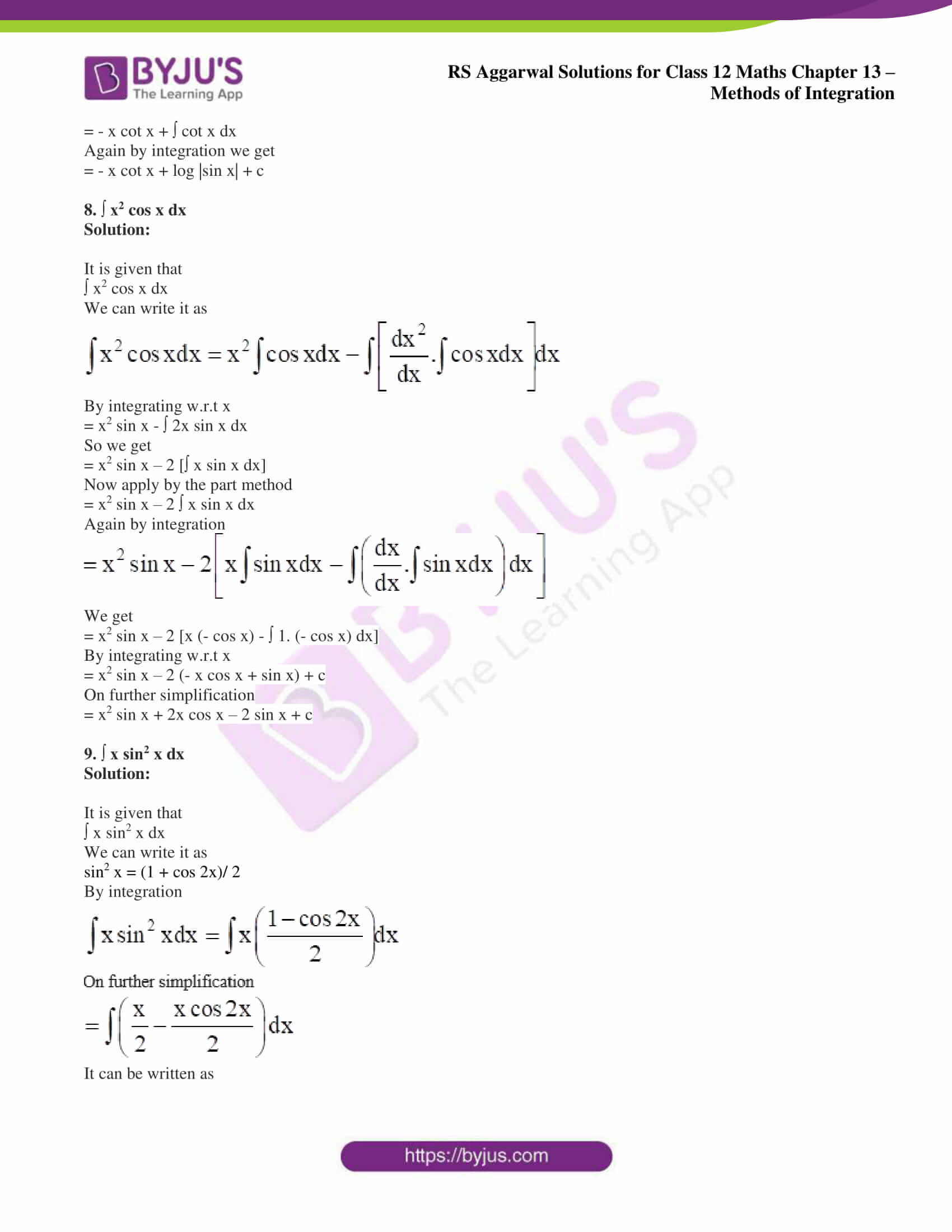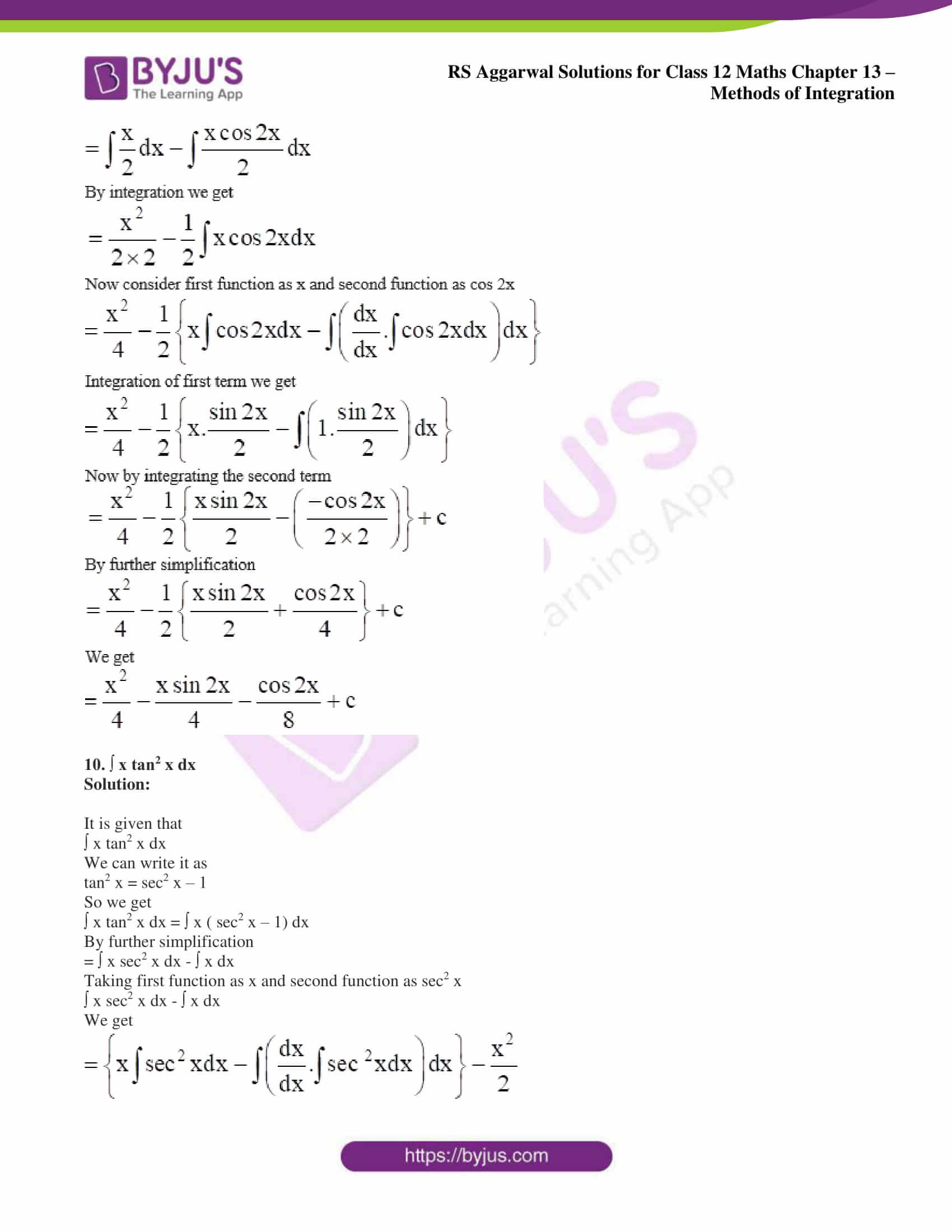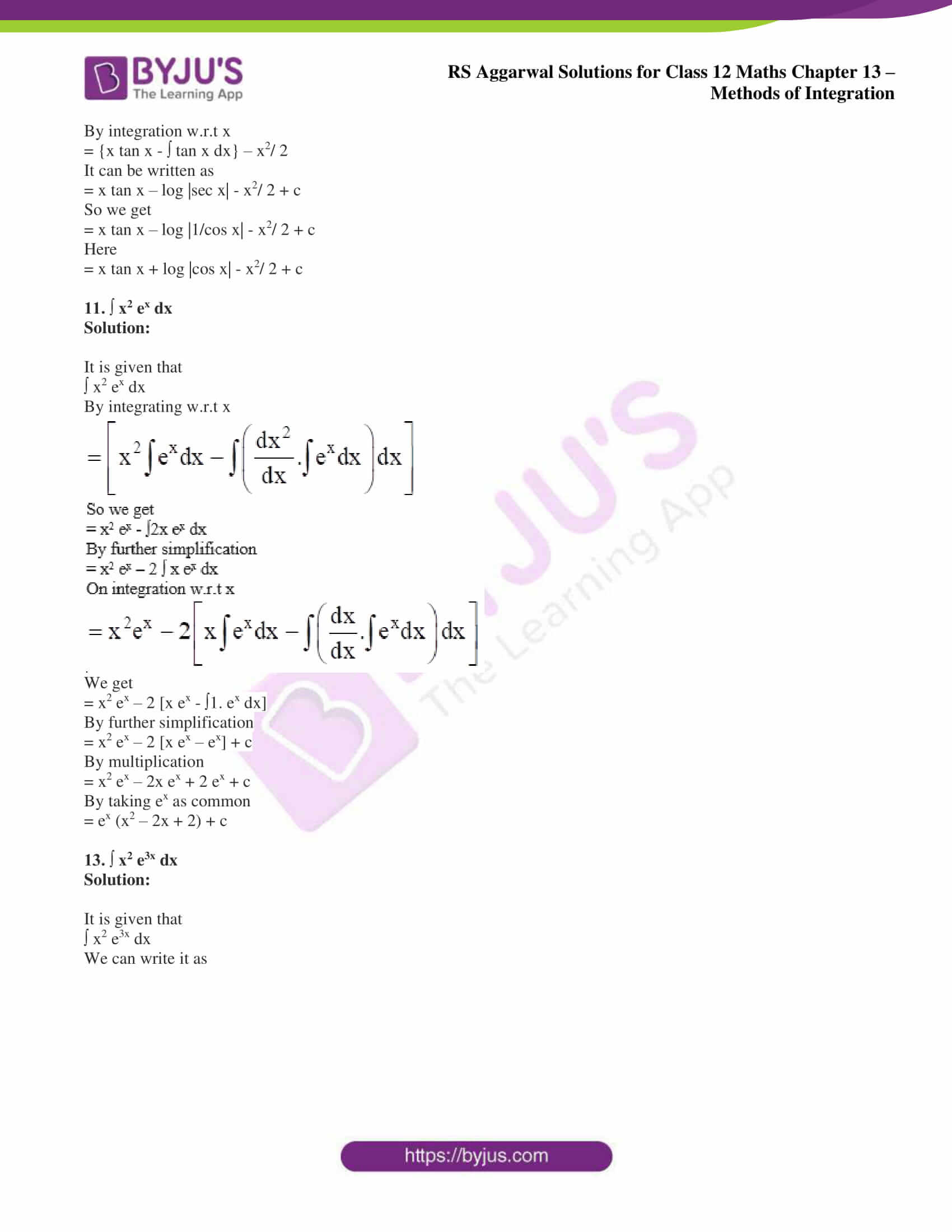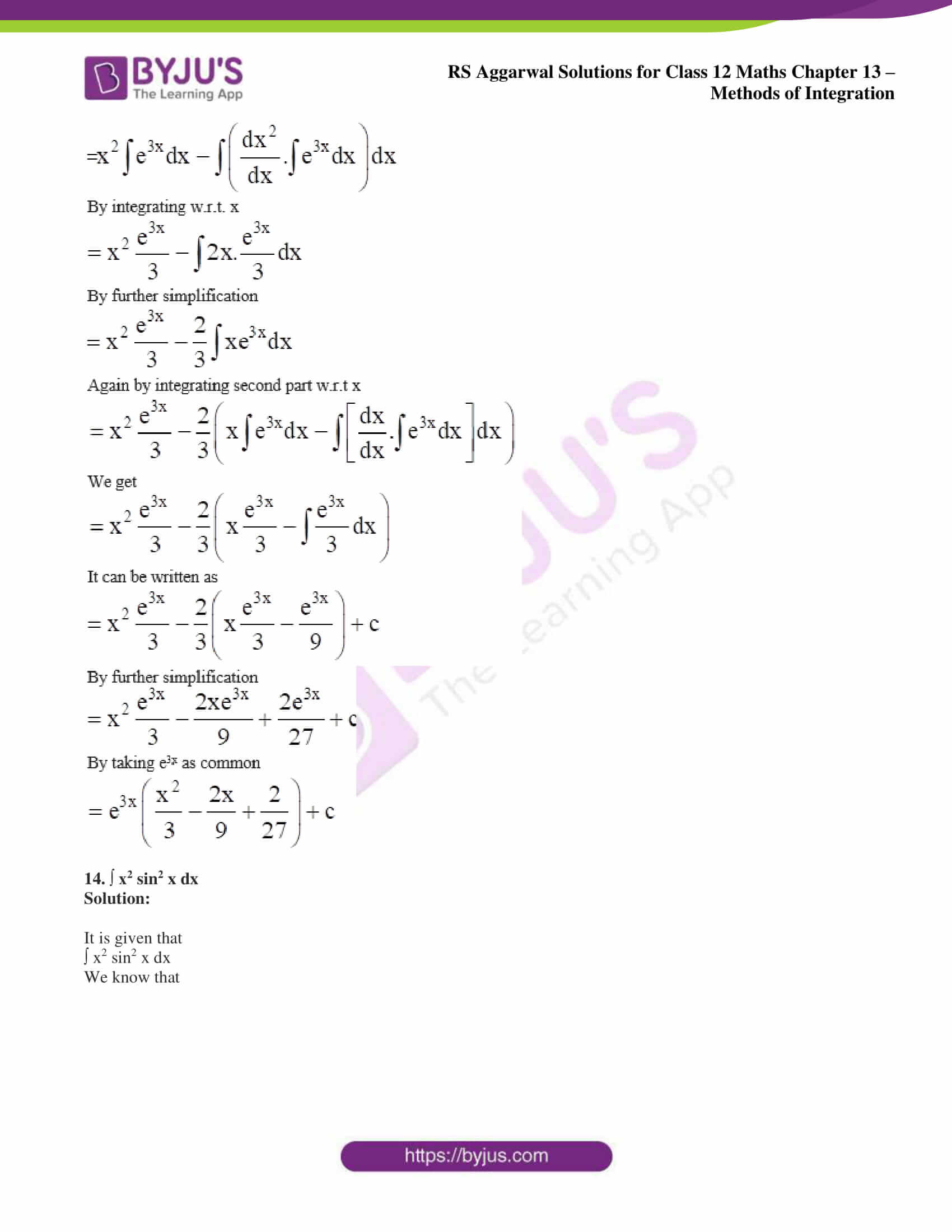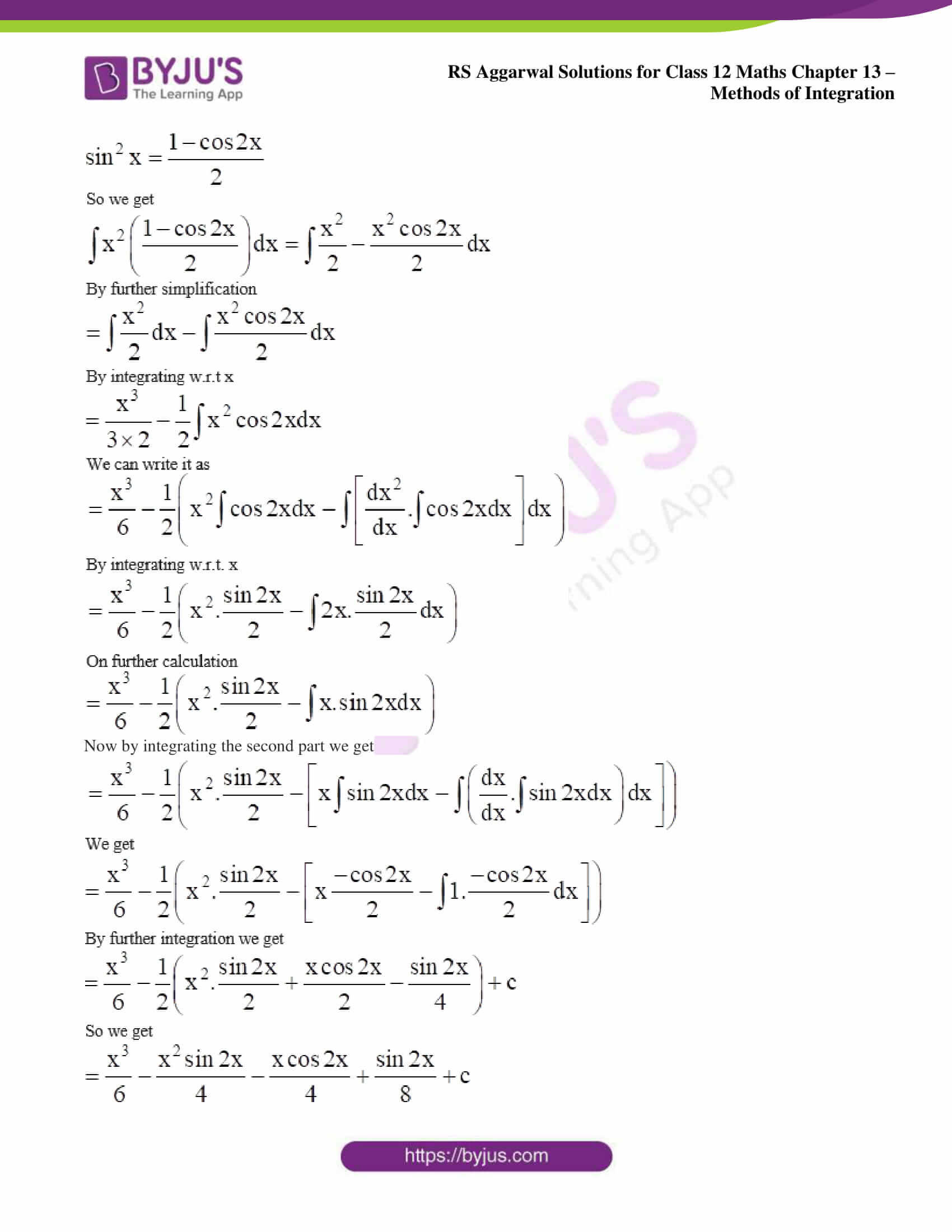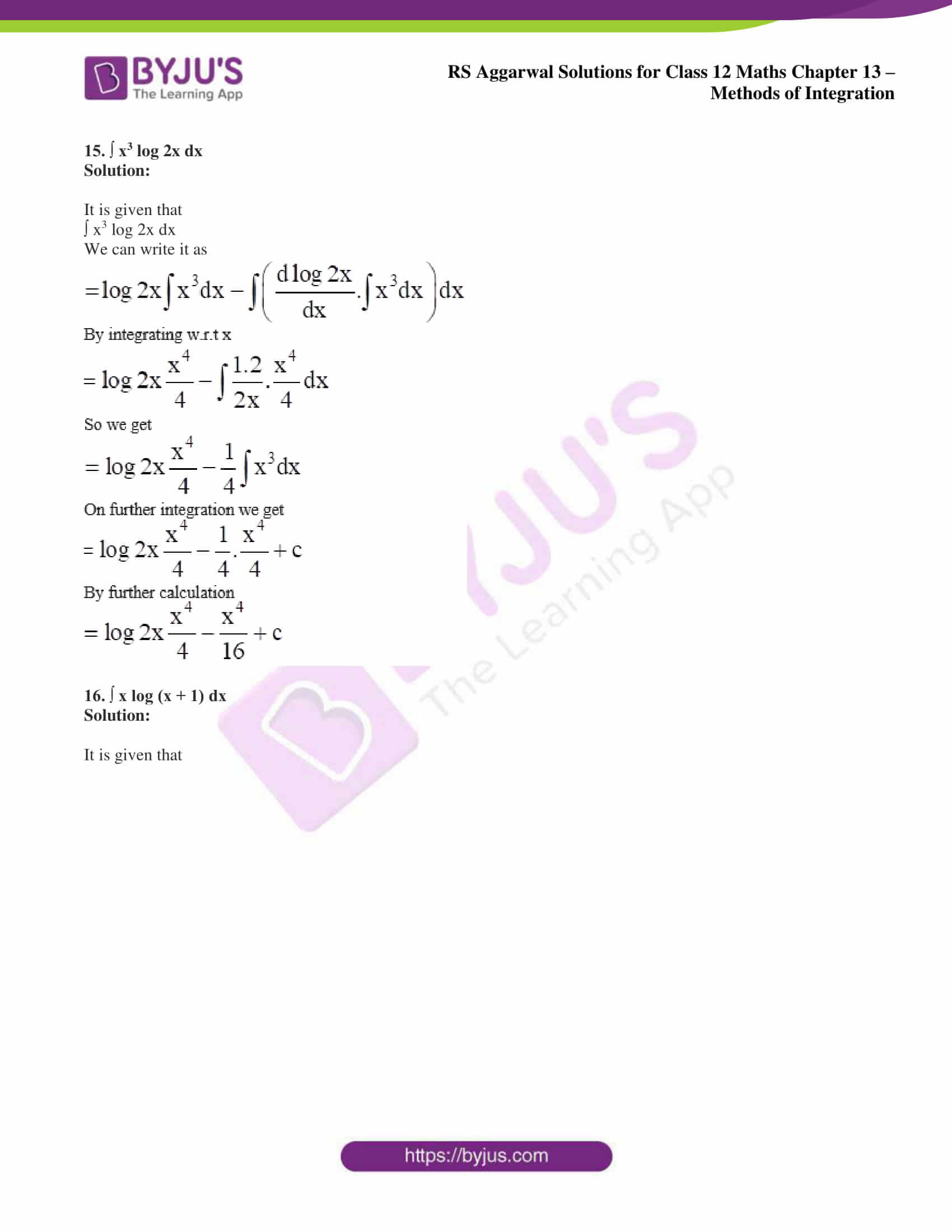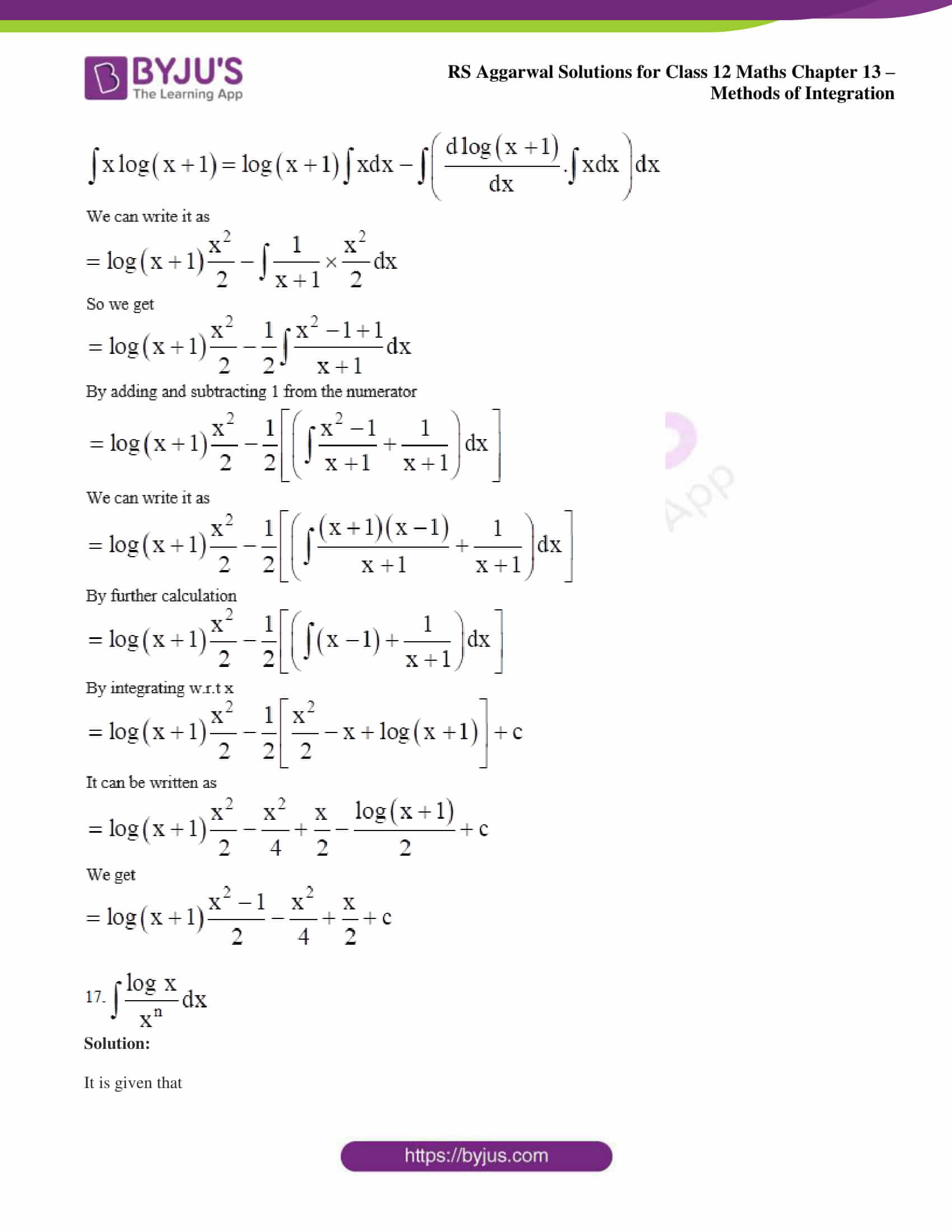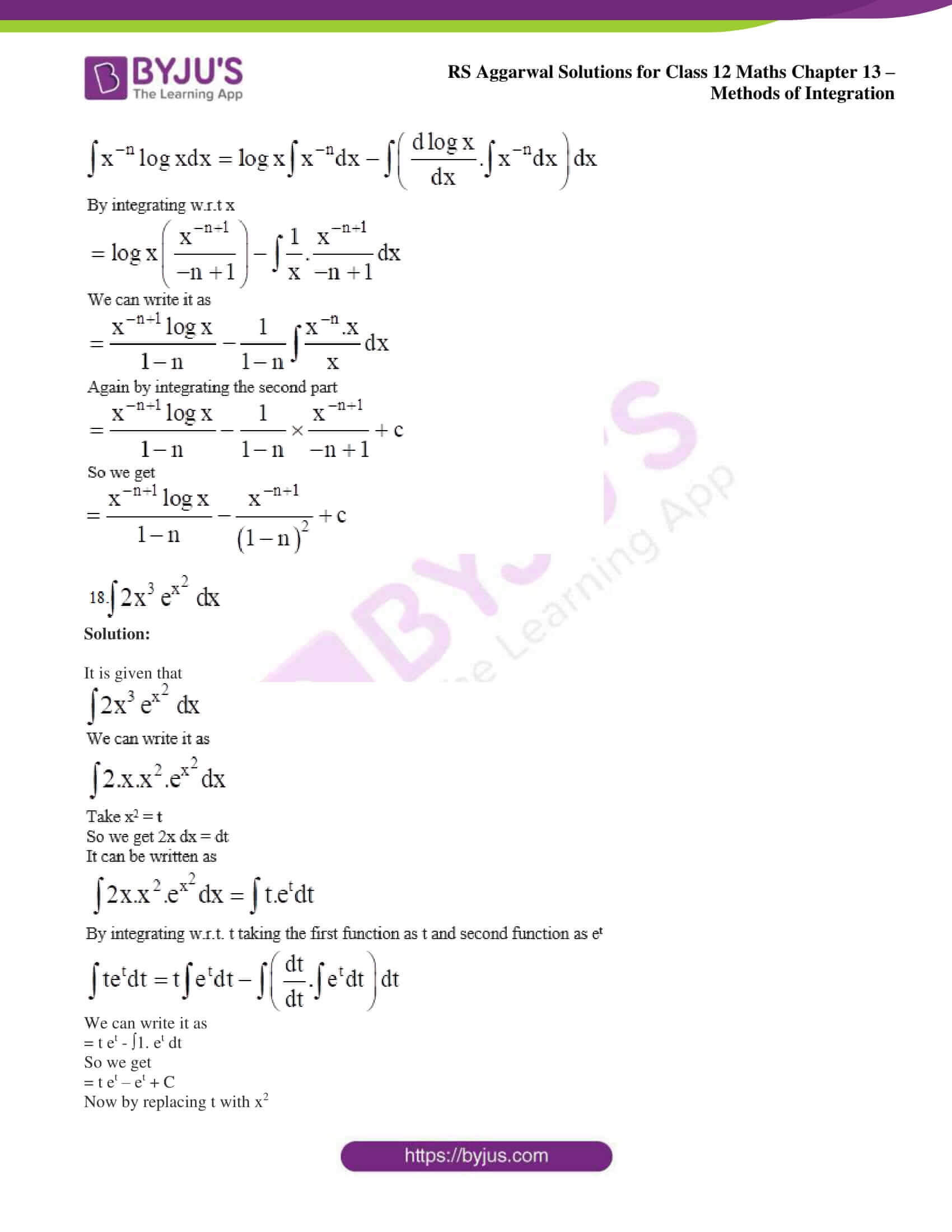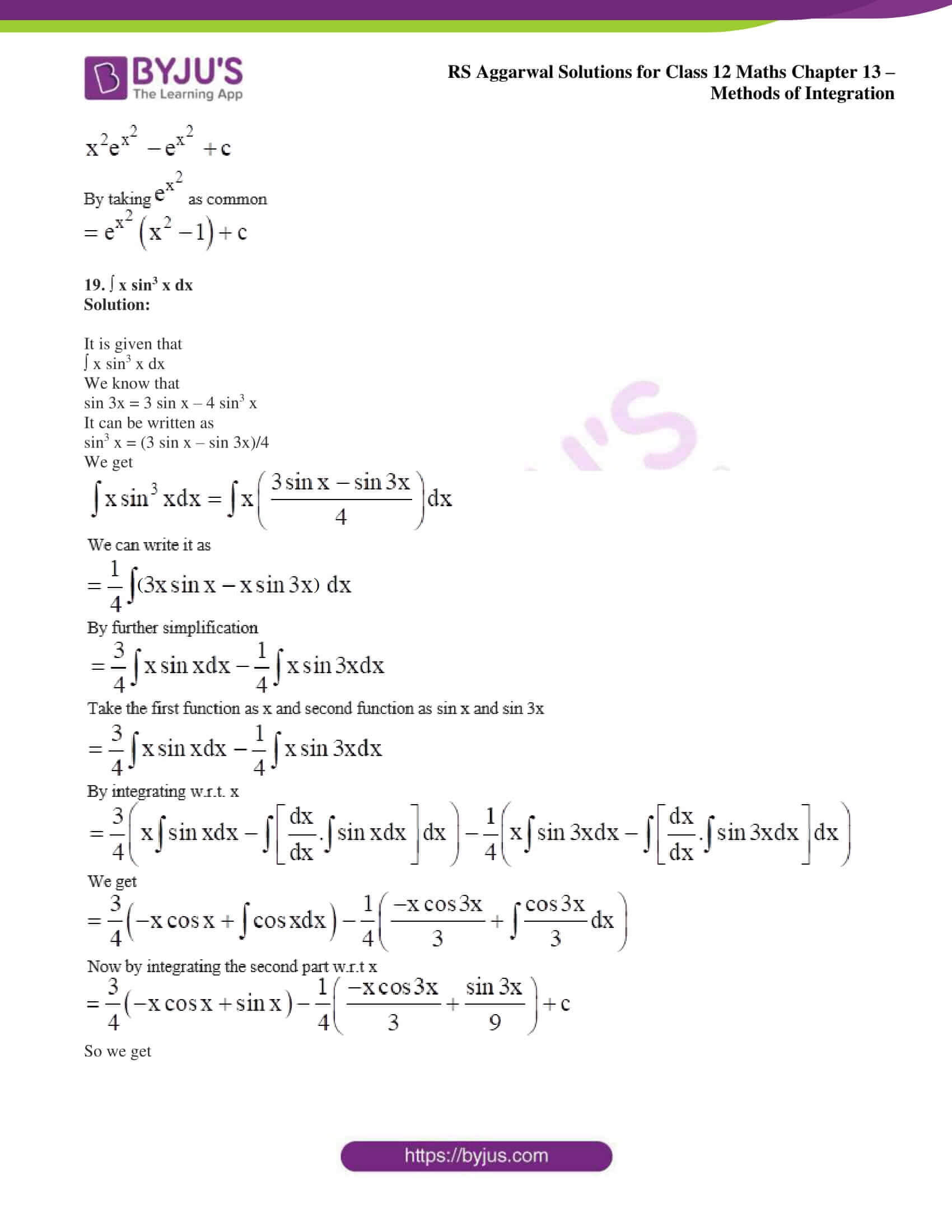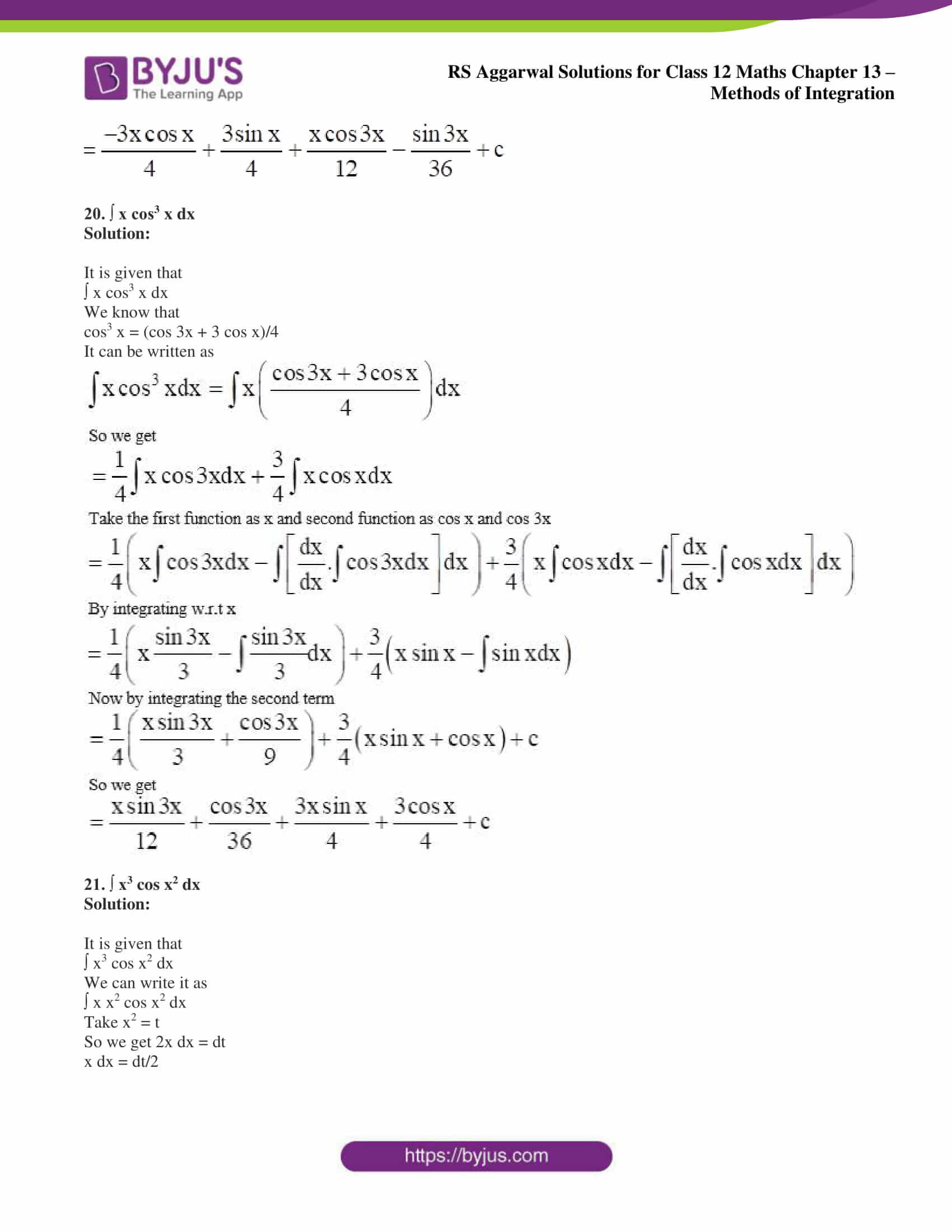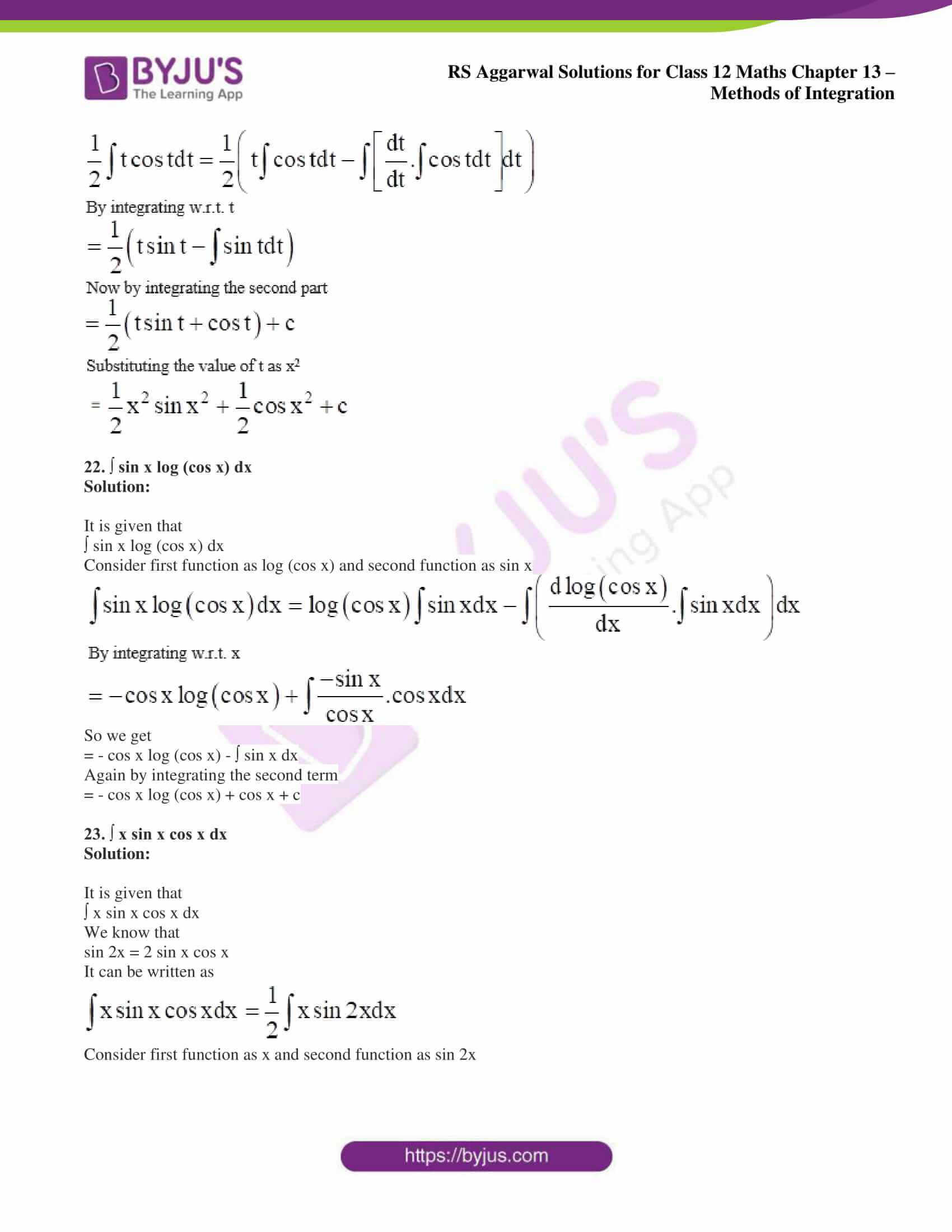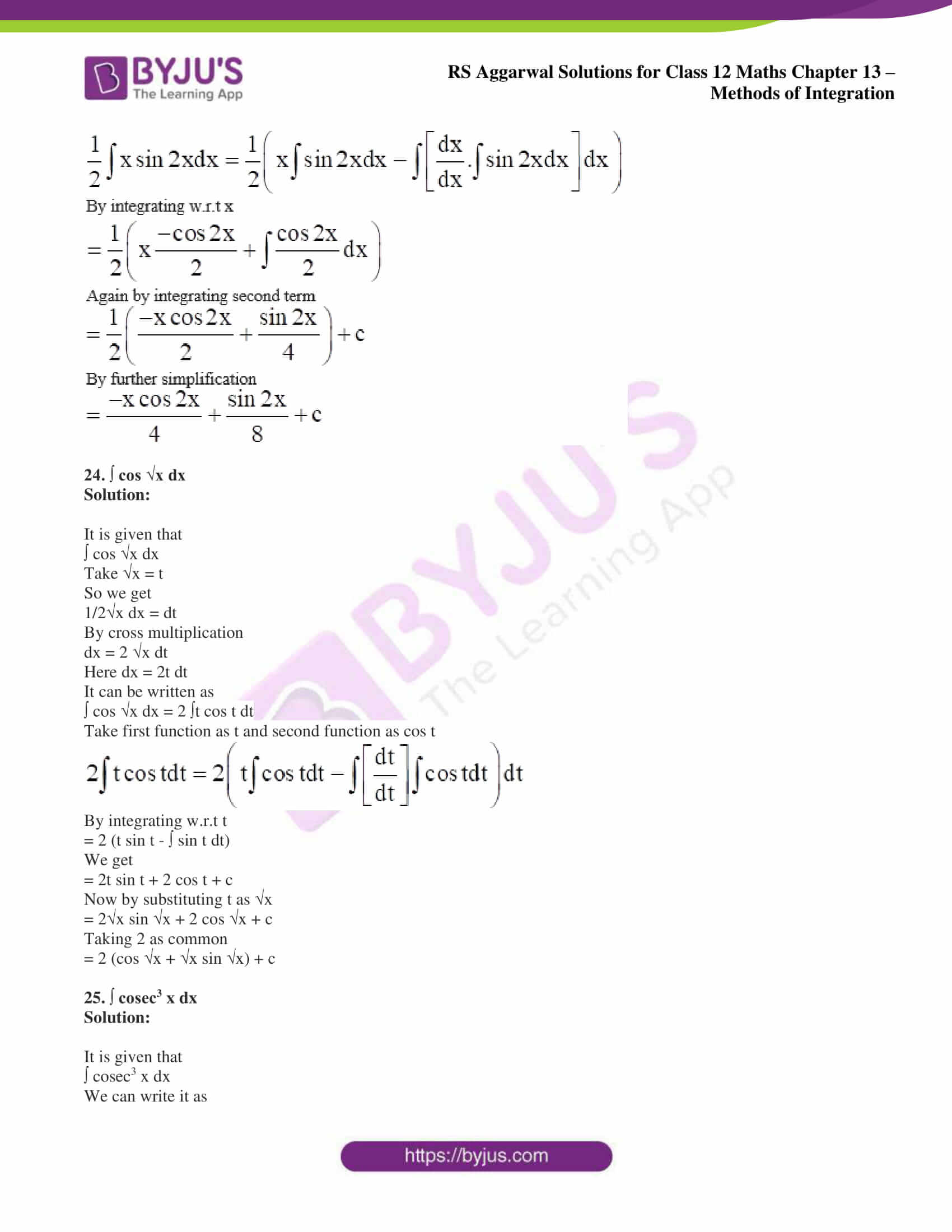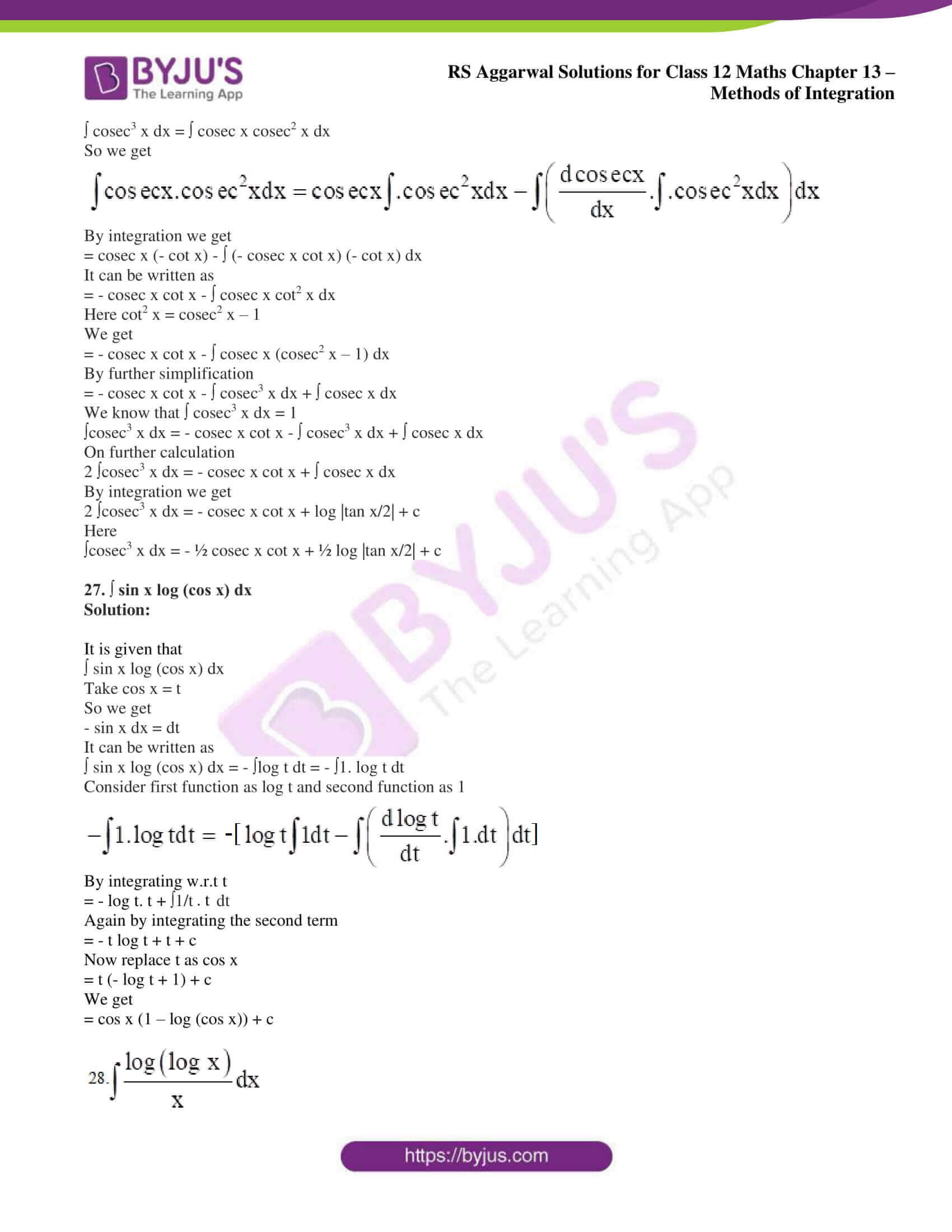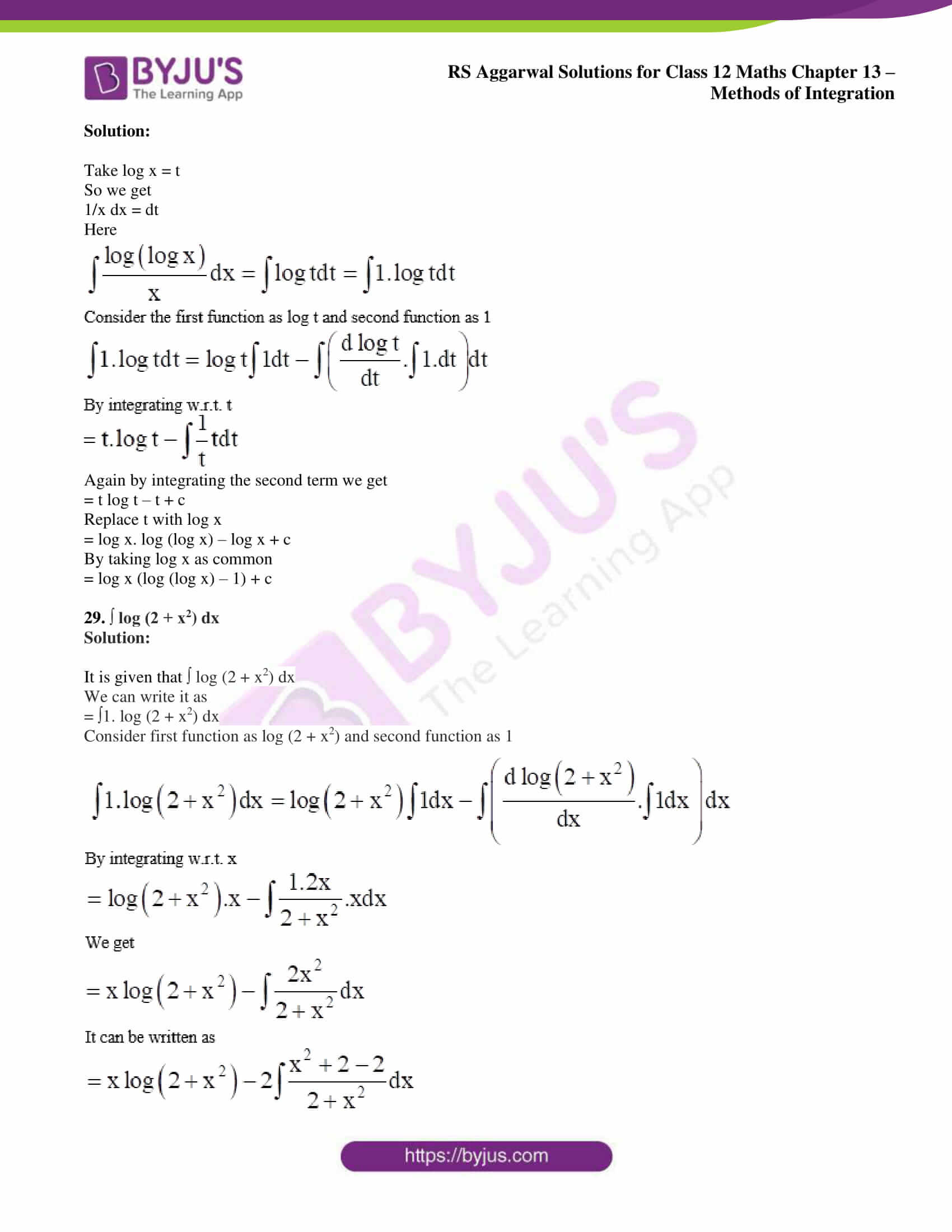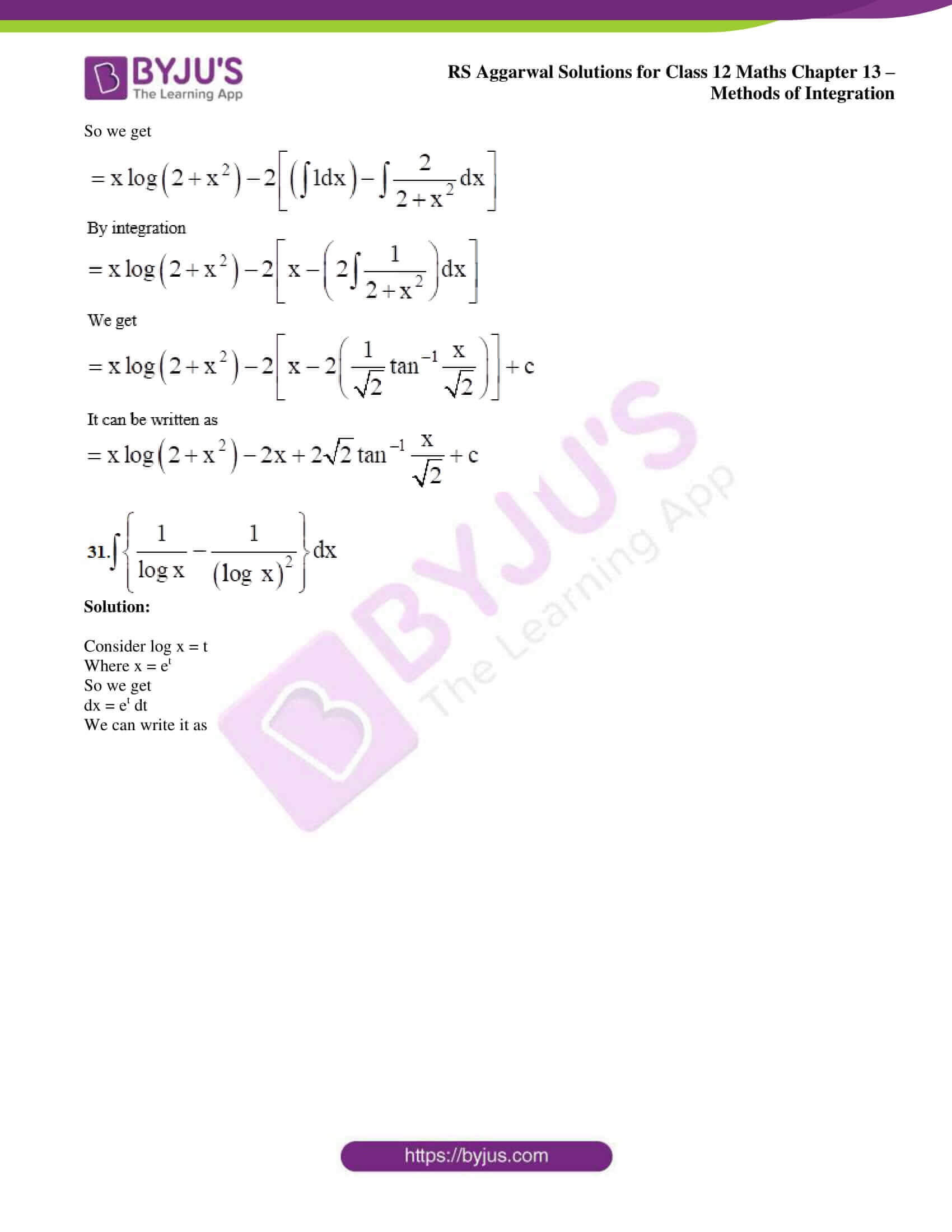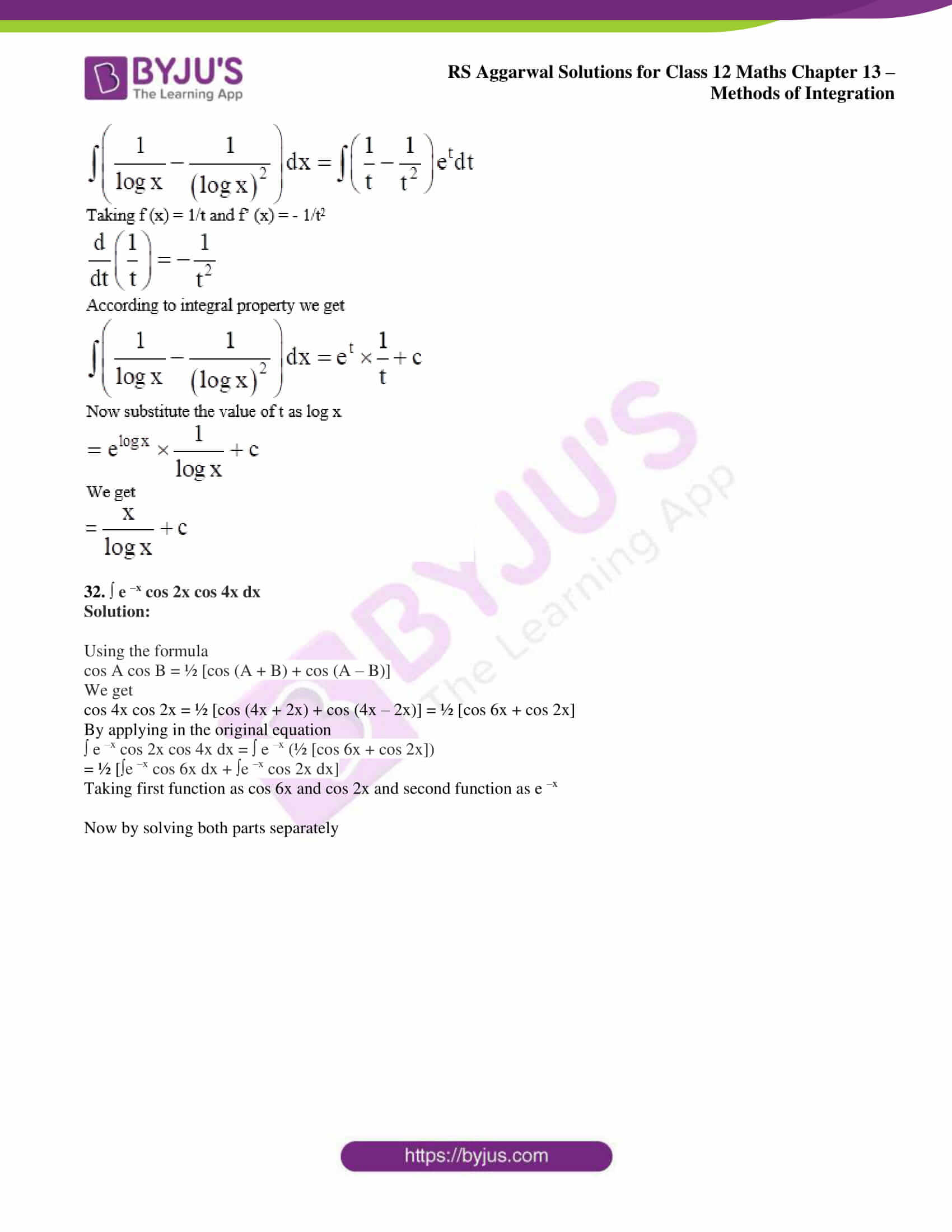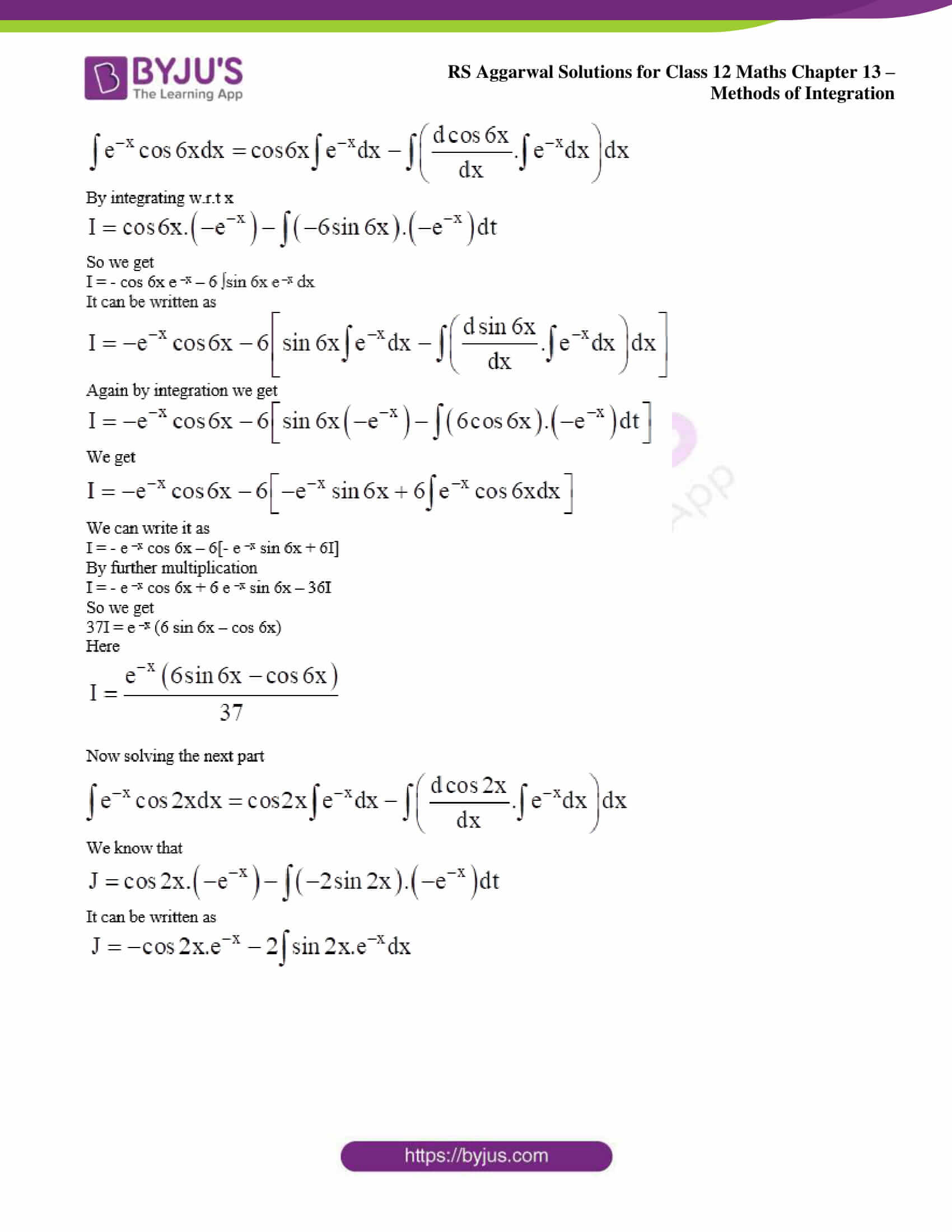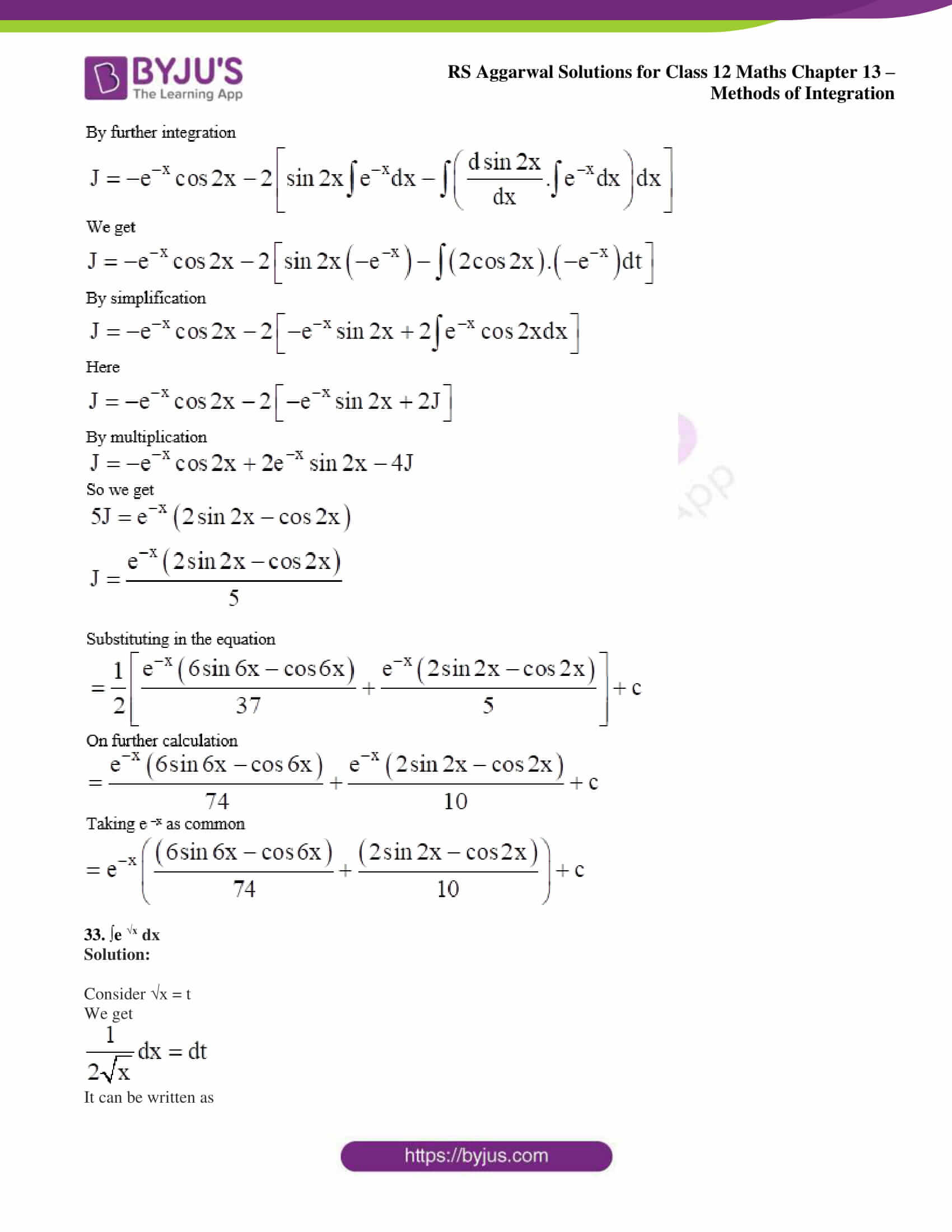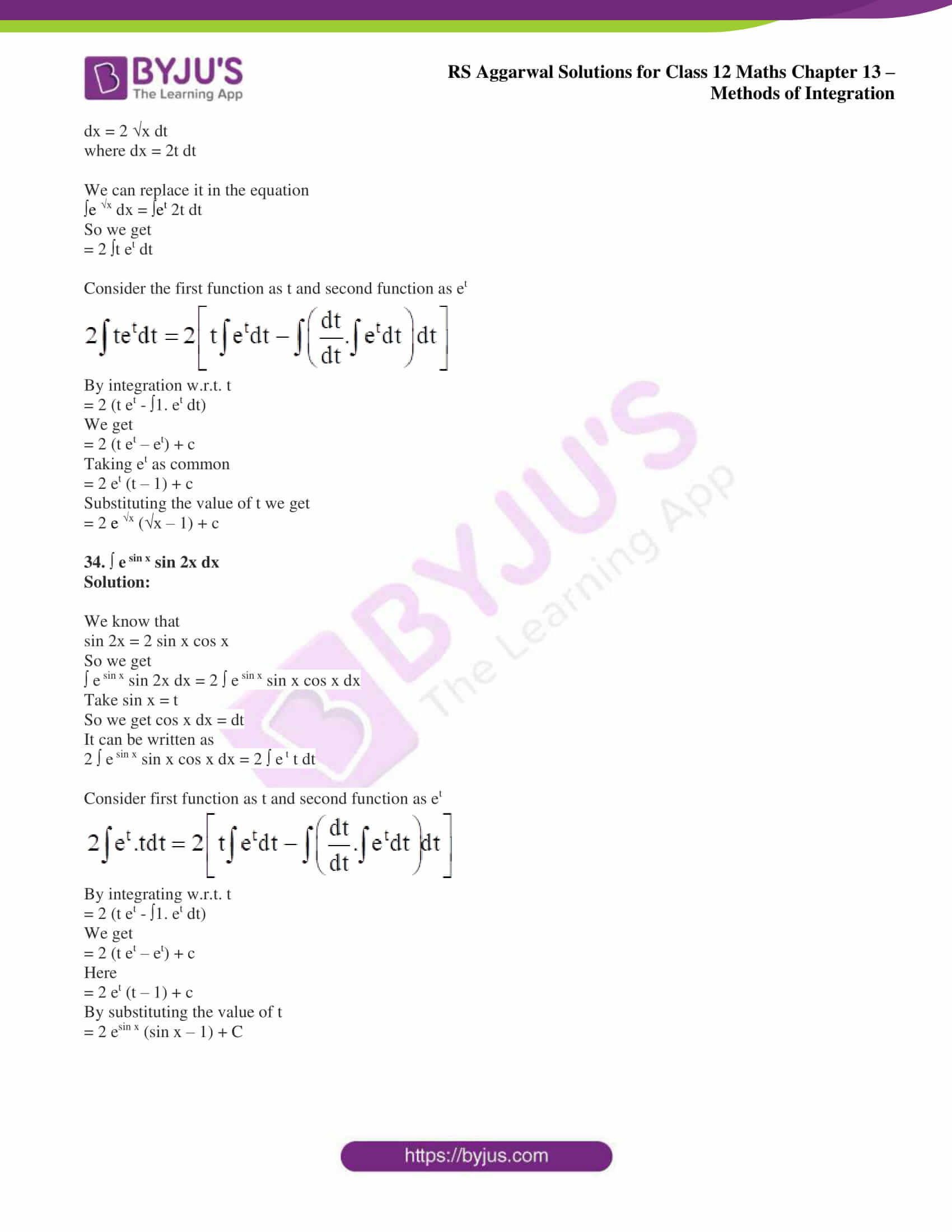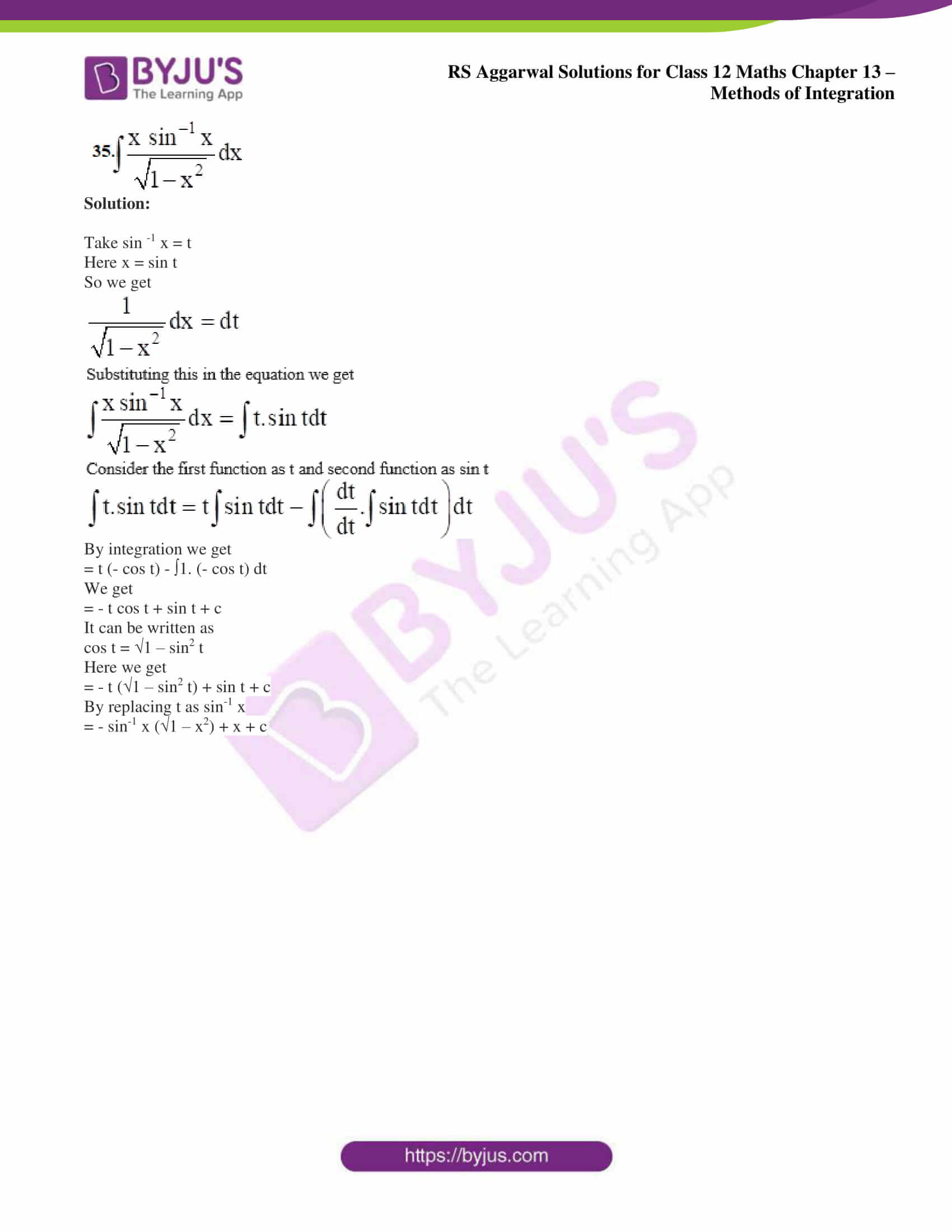### Access other exercise solutions of Class 12 Maths Chapter 13: Methods of Integration

Exercise 13A Solutions

Exercise 13B Solutions

### Access RS Aggarwal Solutions for Class 12 Maths Chapter 13: Methods of Integration Exercise 13C

Evaluate the following integrals:

1. ∫ x ex dx

Solution:

It is given that

∫ x ex dx

We can write it as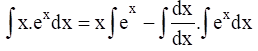By integrating w.r.t x

= x ex – ∫ 1 ex dx

So we get

= x ex – ex + c

By taking ex as common

= ex (x – 1) + c

2. ∫ x cos x dx

Solution:

It is given that

∫ x cos x dx

We can write it as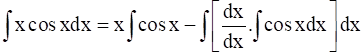By integrating w.r.t x

= x sin x – ∫ 1 sin x dx

So we get

= x sin x + cos x + c

3. ∫ x e2x dx

Solution:

It is given that

∫ x e2x dx

We can write it as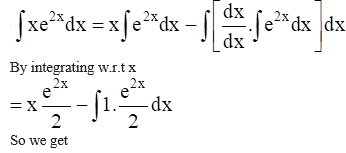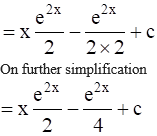4. ∫ x sin 3x dx

Solution:

It is given that

∫ x sin 3x dx

We can write it as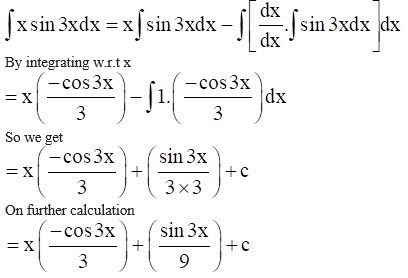5. ∫ x cos 2x dx

Solution:

It is given that

∫ x cos 2x dx

We can write it as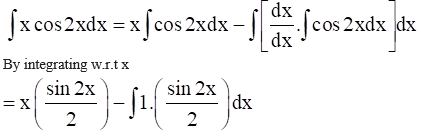So we get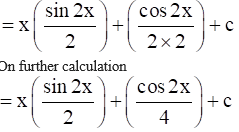6. ∫ x log 2x dx

Solution:

It is given that

∫ x log 2x dx

We can write it as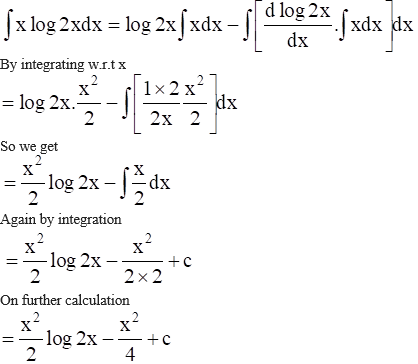7. ∫ x cosec2 x dx

Solution:

It is given that

∫ x cosec2 x dx

We can write it as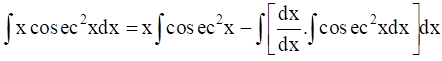By integrating w.r.t x

= x (- cot x) – ∫ 1 (- cot x) dx

So we get

= – x cot x + ∫ cot x dx

Again by integration we get

= – x cot x + log |sin x| + c

8. ∫ x2 cos x dx

Solution:

It is given that

∫ x2 cos x dx

We can write it as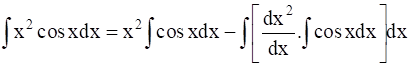By integrating w.r.t x

= x2 sin x – ∫ 2x sin x dx

So we get

= x2 sin x – 2 [∫ x sin x dx]

Now apply by the part method

= x2 sin x – 2 ∫ x sin x dx

Again by integration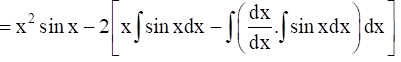We get

= x2 sin x – 2 [x (- cos x) – ∫ 1. (- cos x) dx]

By integrating w.r.t x

= x2 sin x – 2 (- x cos x + sin x) + c

On further simplification

= x2 sin x + 2x cos x – 2 sin x + c

9. ∫ x sin2 x dx

Solution:

It is given that

∫ x sin2 x dx

We can write it as

sin2 x = (1 + cos 2x)/ 2

By integration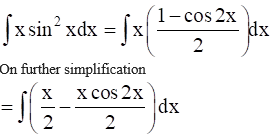It can be written as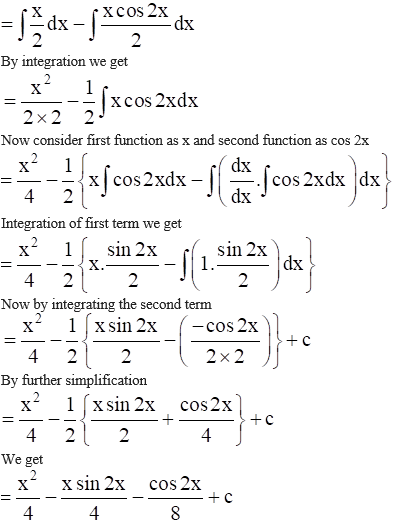10. ∫ x tan2 x dx

Solution:

It is given that

∫ x tan2 x dx

We can write it as

tan2 x = sec2 x – 1

So we get

∫ x tan2 x dx = ∫ x ( sec2 x – 1) dx

By further simplification

= ∫ x sec2 x dx – ∫ x dx

Taking first function as x and second function as sec2 x

∫ x sec2 x dx – ∫ x dx

We get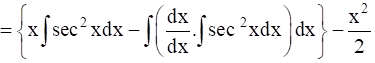By integration w.r.t x

= {x tan x – ∫ tan x dx} – x2/ 2

It can be written as

= x tan x – log |sec x| – x2/ 2 + c

So we get

= x tan x – log |1/cos x| – x2/ 2 + c

Here

= x tan x + log |cos x| – x2/ 2 + c

11. ∫ x2 ex dx

Solution:

It is given that

∫ x2 ex dx

By integrating w.r.t x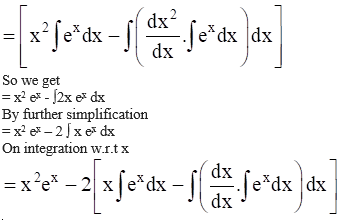We get

= x2 ex – 2 [x ex – ∫1. ex dx]

By further simplification

= x2 ex – 2 [x ex – ex] + c

By multiplication

= x2 ex – 2x ex + 2 ex + c

By taking ex as common

= ex (x2 – 2x + 2) + c

13. ∫ x2 e3x dx

Solution:

It is given that

∫ x2 e3x dx

We can write it as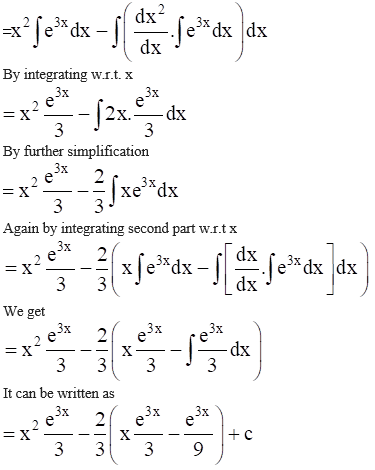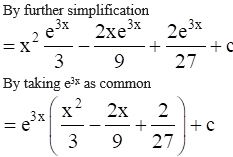14. ∫ x2 sin2 x dx

Solution:

It is given that

∫ x2 sin2 x dx

We know that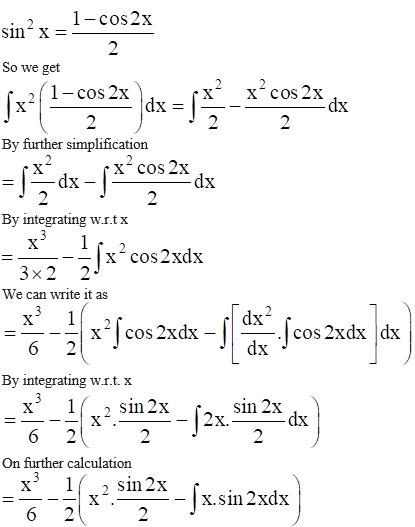Now by integrating the second part we get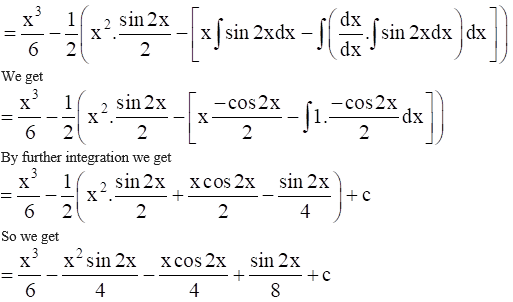15. ∫ x3 log 2x dx

Solution:

It is given that

∫ x3 log 2x dx

We can write it as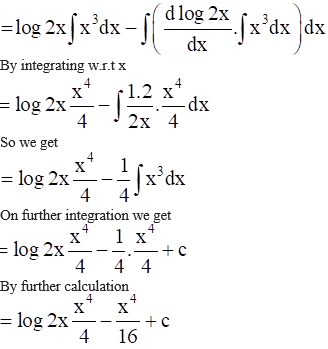16. ∫ x log (x + 1) dx

Solution:

It is given that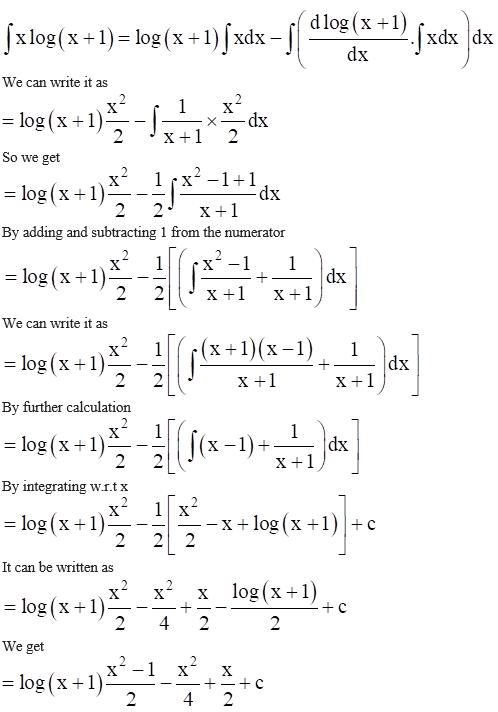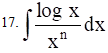Solution:

It is given that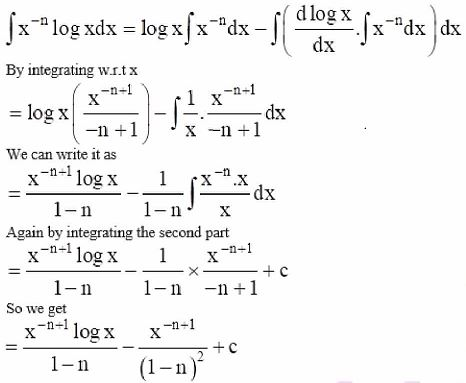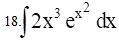Solution:

It is given that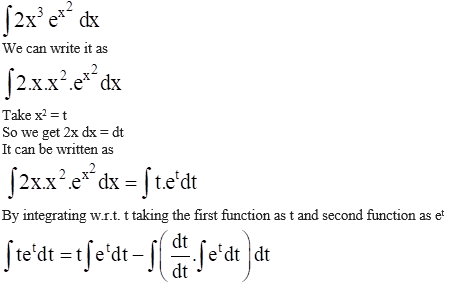We can write it as

= t et – ∫1. et dt

So we get

= t et – et + C

Now by replacing t with x2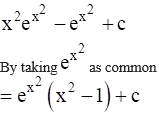19. ∫ x sin3 x dx

Solution:

It is given that

∫ x sin3 x dx

We know that

sin 3x = 3 sin x – 4 sin3 x

It can be written as

sin3 x = (3 sin x – sin 3x)/4

We get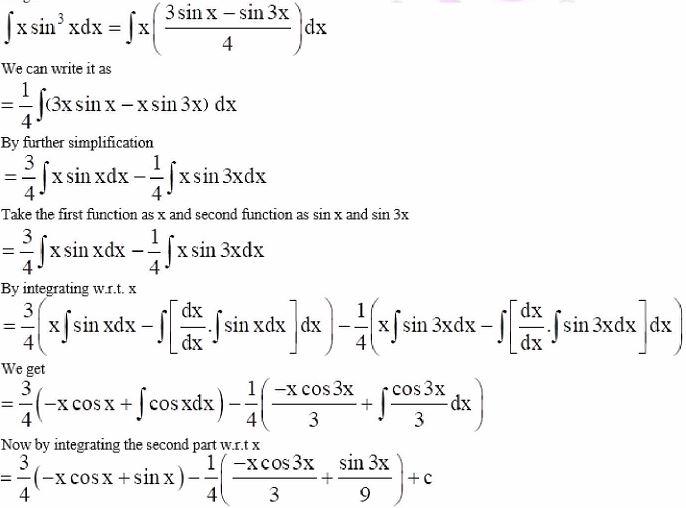So we get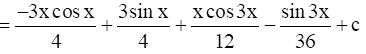20. ∫ x cos3 x dx

Solution:

It is given that

∫ x cos3 x dx

We know that

cos3 x = (cos 3x + 3 cos x)/4

It can be written as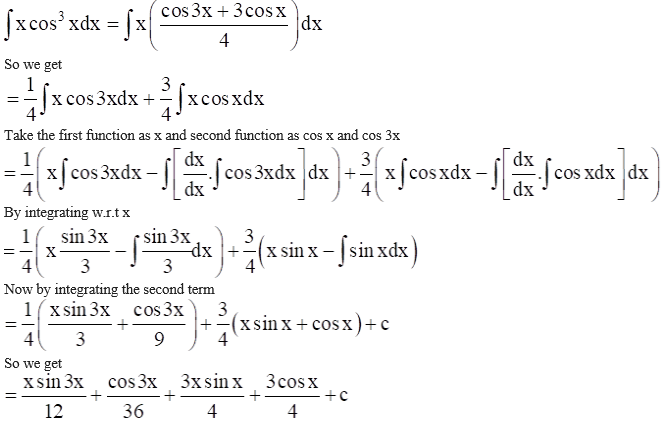21. ∫ x3 cos x2 dx

Solution:

It is given that

∫ x3 cos x2 dx

We can write it as

∫ x x2 cos x2 dx

Take x2 = t

So we get 2x dx = dt

x dx = dt/2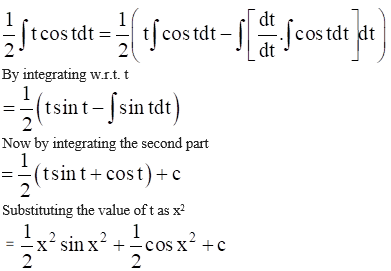22. ∫ sin x log (cos x) dx

Solution:

It is given that

∫ sin x log (cos x) dx

Consider first function as log (cos x) and second function as sin x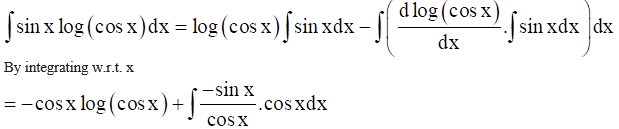So we get

= – cos x log (cos x) – ∫ sin x dx

Again by integrating the second term

= – cos x log (cos x) + cos x + c

23. ∫ x sin x cos x dx

Solution:

It is given that

∫ x sin x cos x dx

We know that

sin 2x = 2 sin x cos x

It can be written as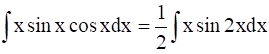Consider first function as x and second function as sin 2x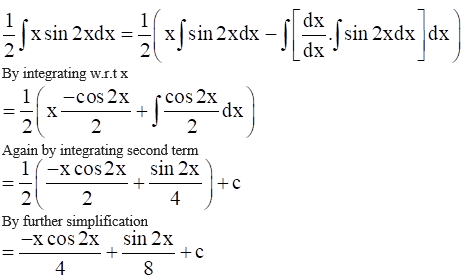24. ∫ cos √x dx

Solution:

It is given that

∫ cos √x dx

Take √x = t

So we get

1/2√x dx = dt

By cross multiplication

dx = 2 √x dt

Here dx = 2t dt

It can be written as

∫ cos √x dx = 2 ∫t cos t dt

Take first function as t and second function as cos t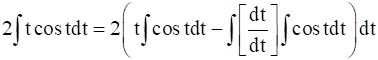By integrating w.r.t t

= 2 (t sin t – ∫ sin t dt)

We get

= 2t sin t + 2 cos t + c

Now by substituting t as √x

= 2√x sin √x + 2 cos √x + c

Taking 2 as common

= 2 (cos √x + √x sin √x) + c

25. ∫ cosec3 x dx

Solution:

It is given that

∫ cosec3 x dx

We can write it as

∫ cosec3 x dx = ∫ cosec x cosec2 x dx

So we get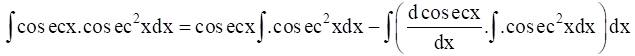By integration we get

= cosec x (- cot x) – ∫ (- cosec x cot x) (- cot x) dx

It can be written as

= – cosec x cot x – ∫ cosec x cot2 x dx

Here cot2 x = cosec2 x – 1

We get

= – cosec x cot x – ∫ cosec x (cosec2 x – 1) dx

By further simplification

= – cosec x cot x – ∫ cosec3 x dx + ∫ cosec x dx

We know that ∫ cosec3 x dx = 1

∫cosec3 x dx = – cosec x cot x – ∫ cosec3 x dx + ∫ cosec x dx

On further calculation

2 ∫cosec3 x dx = – cosec x cot x + ∫ cosec x dx

By integration we get

2 ∫cosec3 x dx = – cosec x cot x + log |tan x/2| + c

Here

∫cosec3 x dx = – ½ cosec x cot x + ½ log |tan x/2| + c

27. ∫ sin x log (cos x) dx

Solution:

It is given that

∫ sin x log (cos x) dx

Take cos x = t

So we get

– sin x dx = dt

It can be written as

∫ sin x log (cos x) dx = – ∫log t dt = – ∫1. log t dt

Consider first function as log t and second function as 1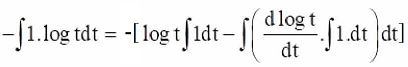By integrating w.r.t t

= – log t. t + ∫1/t. t dt

Again by integrating the second term

= – t log t + t + c

Now replace t as cos x

= t (- log t + 1) + c

We get

= cos x (1 – log (cos x)) + c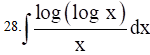Solution:

Take log x = t

So we get

1/x dx = dt

Here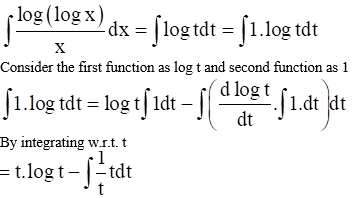Again by integrating the second term we get

= t log t – t + c

Replace t with log x

= log x. log (log x) – log x + c

By taking log x as common

= log x (log (log x) – 1) + c

29. ∫ log (2 + x2) dx

Solution:

It is given that ∫ log (2 + x2) dx

We can write it as

= ∫1. log (2 + x2) dx

Consider first function as log (2 + x2) and second function as 1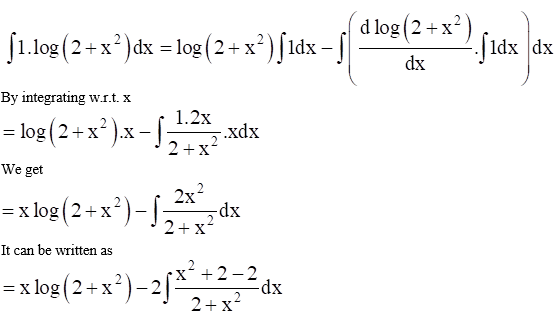So we get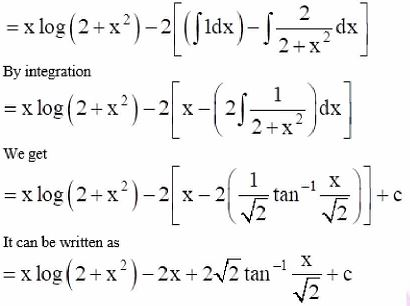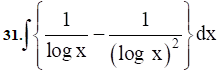Solution:

Consider log x = t

Where x = et

So we get

dx = et dt

We can write it as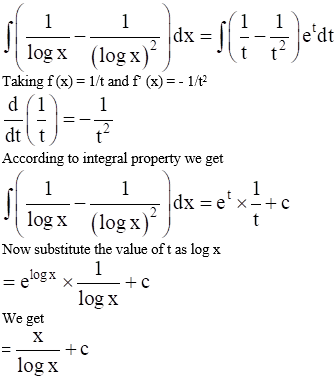32. ∫ e –x cos 2x cos 4x dx

Solution:

Using the formula

cos A cos B = ½ [cos (A + B) + cos (A – B)]

We get

cos 4x cos 2x = ½ [cos (4x + 2x) + cos (4x – 2x)] = ½ [cos 6x + cos 2x]

By applying in the original equation

∫ e –x cos 2x cos 4x dx = ∫ e –x (½ [cos 6x + cos 2x])

= ½ [∫e –x cos 6x dx + ∫e –x cos 2x dx]

Taking first function as cos 6x and cos 2x and second function as e –x

Now by solving both parts separately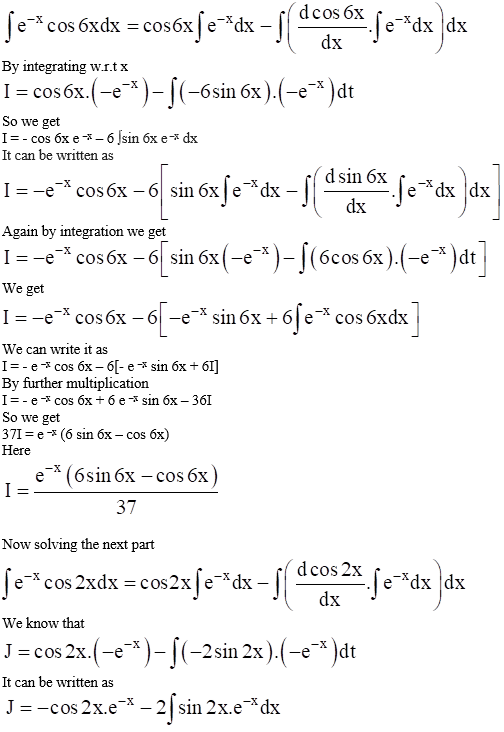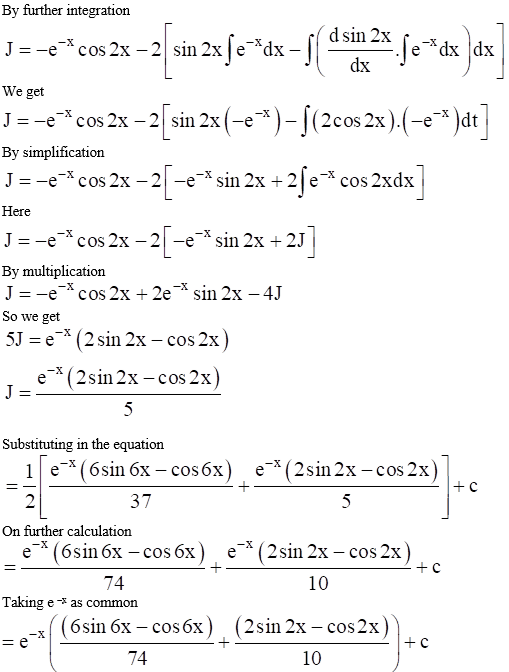33. ∫e √x dx

Solution:

Consider √x = t

We get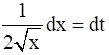It can be written as

dx = 2 √x dt

where dx = 2t dt

We can replace it in the equation

∫e √x dx = ∫et 2t dt

So we get

= 2 ∫t et dt

Consider the first function as t and second function as et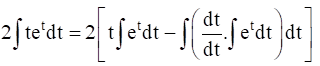By integration w.r.t. t

= 2 (t et – ∫1. et dt)

We get

= 2 (t et – et) + c

Taking et as common

= 2 et (t – 1) + c

Substituting the value of t we get

= 2 e √x (√x – 1) + c

34. ∫ e sin x sin 2x dx

Solution:

We know that

sin 2x = 2 sin x cos x

So we get

∫ e sin x sin 2x dx = 2 ∫ e sin x sin x cos x dx

Take sin x = t

So we get cos x dx = dt

It can be written as

2 ∫ e sin x sin x cos x dx = 2 ∫ e t t dt

Consider first function as t and second function as et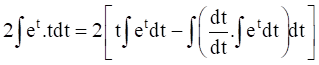By integrating w.r.t. t

= 2 (t et – ∫1. et dt)

We get

= 2 (t et – et) + c

Here

= 2 et (t – 1) + c

By substituting the value of t

= 2 esin x (sin x – 1) + C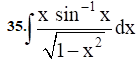Solution:

Take sin -1 x = t

Here x = sin t

So we get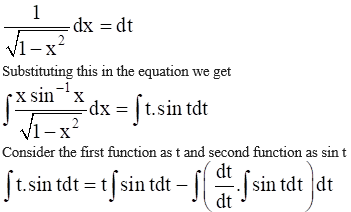By integration we get

= t (- cos t) – ∫1. (- cos t) dt

We get

= – t cos t + sin t + c

It can be written as

cos t = √1 – sin2 t

Here we get

= – t (√1 – sin2 t) + sin t + c

By replacing t as sin-1 x

= – sin-1 x (√1 – x2) + x + c